# How to Compare Two Columns and Highlight Duplicate Values in Excel

Suppose we have two lists, list A contains some products serial numbers, list B also contains some products serial numbers, and some of them are found in list A as well. So, there are some duplicate values in list A and list B. If we want to find them out, we can though compare the two list to find out the duplicate values. Actually, in our work we often meet the situations that we need to compare two lists and find out the duplicate values. In this tutorial, we will help you to fix this issue by two ways, the first one, we can use Conditional Formatting function in excel; the second one, we can use formulas.

Precondition:

Prepare two columns. Column A and column B list product serial number. There are some duplicate values.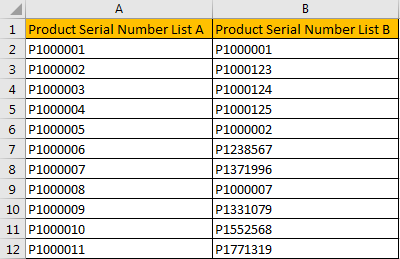## Method 1: Compare Two Columns and Highlight Duplicate Values by Conditional Formatting Function

Step 1: Select List A and List B.

Step 2: Click Home in ribbon, click Conditional Formatting in Styles group.Step 3: In Conditional Formatting dropdown list, select Highlight Cells Rules->Duplicate Values.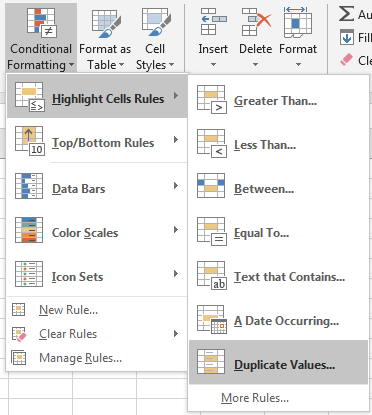Step 4: In Duplicate Values dialog, select Duplicate in dropdown list. Keep default value in values with dropdown list. Then click OK.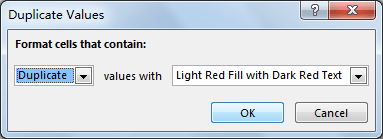Step 5: Verify that all duplicate values in both column A and column B are marked with dark red properly.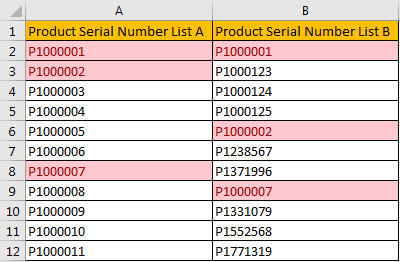## Method 2: Compare Two Columns and Highlight Duplicate Values by Formulas

Step 1: Insert a new column between column A and column B. Then in B2, enter the formula =ISNA(VLOOKUP(A2,\$C\$2:\$C\$12,1,FALSE)). Click Enter, and we get FALSE.

In this formula, we can through VLOOKUP function to know whether value in column A exists in column B, if yes, VLOOKUP function will return matching value, otherwise it will return blank value, then we can use ISNA function (check whether a value is N/A) to get TRUE or FALSE value finally.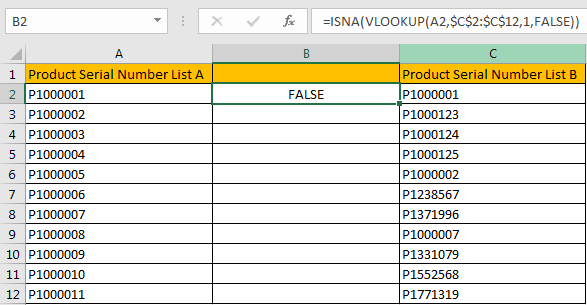Step 2: Drag the fill handle down. Verify that B3:B12 are filled with TRUE or FALSE accordingly.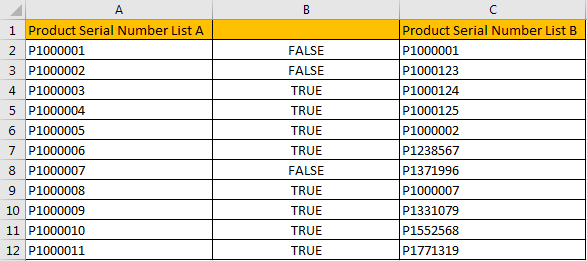Step 3: Create a filter on column B. Click Data in ribbon, select Filter in Sort & Filter group.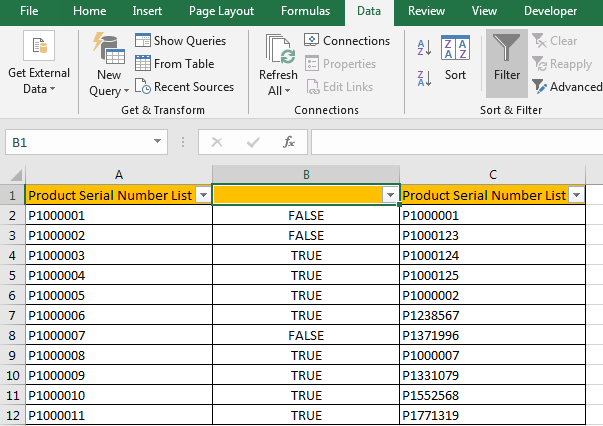Step 4: Click filter arrow in B1. Only check on FALSE, click OK.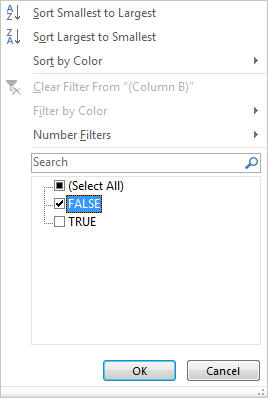Step 5: After above settings, duplicate values are filtered. Fill them with yellow.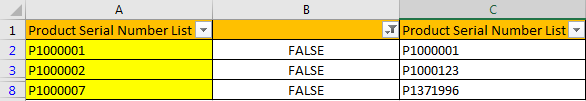Step 6: Clear column B, then in B2 enter the formula =ISNA(VLOOKUP(C2,\$A\$2:\$A\$12,1,FALSE)).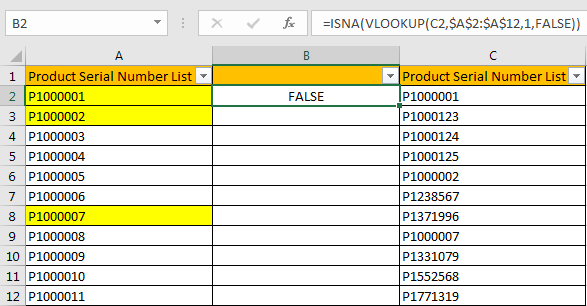Step 7: Repeat above steps#2-#5 to highlight duplicate values in column C.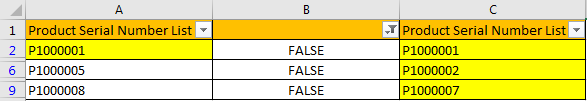Step 8: Clear filter in column B. And delete column B. Verify that all duplicate values are highlighted.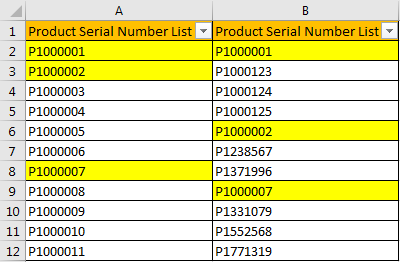Comment:

We can also use COUNTIF function here. Enter =COUNTIF(\$C\$2:\$C\$12,A2) in B1, then we can get 1 for duplicate values or 0 for unique values.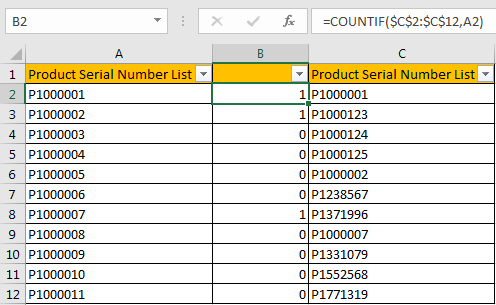Related Posts

Cap percentage values between 0 and 100

This article will talk about how to cap the percentage values between 0% and 100% in Microsoft Excel Spreadsheet or Google Sheets. If you are a newbie on Excel or google Sheets, you may be able to do this by ...

Calculate Cap Percentages to Specific Value

This article will talk about how to limit the cap percentage of a given amount to a given value in Microsoft Excel Spreadsheet or Google Sheets. If you are a newbie on Excel or google Sheets, you may be able ...

Calculate Win Loss Tie

Suppose you got a task to calculate the win, loss, and tie totals; what would you do? If you are new to Ms Excel and don't have enough experience with it, then you might do this task manually but let ...

Calculate Years Between Dates In Ms Excel

If you are an avid Ms Excel user, then you might have come across a task in which you needed to calculate the years between the dates; you might take it easy and do this task manually, which is also ...

Calculate Number of Hours between Two Times

Calculating the difference between two times might be a valuable statistic for subsequent computations or averages, whether you're producing a time sheet for staff or recording personal exercises. While Excel has a plethora of complex functions, including date and time ...

Calculate Loan Interest in Given Year

When you borrow money, you are supposed to repay it gradually. Lenders, on the other hand, want to be compensated for their services and the risk they incur by lending you money. That is, you will not just repay the ...

Calculate Interest Rate for Loan

The interest rate is the fee charged by a lender to a borrower and is expressed as a percentage of the principal—the lent amount. The interest rate on a loan is often expressed as an annual percentage rate, abbreviated as ...

Calculate Interest for Given Period

Using the IPMT function in Excel, we can compute the interest payment on any loan. This step-by-step tutorial will guide Excel users of all skill levels through the process to calculate interest for given period. Finally, the formula: =IPMT(B3/12,1,B5,-B2) The ...

How To Use Excel GCD Function

This post will guide you how to use Excel GCD function with syntax and examples in Microsoft excel. Description The Excel GCD function Returns the greatest common divisor of two or more integers. So you can use the GCD function ...

Calculate A Ratio From Two Numbers In Excel

In elementary mathematics, a ratio is a connection or comparison between two or more integers. For example, ratios are often expressed as ":" to demonstrate the relationship between two numbers. You would think that manually calculating a ratio from two ...

Sidebar